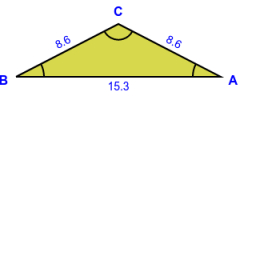# Calculate 71764

The angle at the base of an isosceles triangle is 18 ° larger than the angle at the central vertex. Calculate the size of the interior angles.

A =  66 °
B =  66 °
C =  48 °

### Step-by-step explanation:Did you find an error or inaccuracy? Feel free to write us. Thank you!

Tips for related online calculators
Do you have a linear equation or system of equations and looking for its solution? Or do you have a quadratic equation?